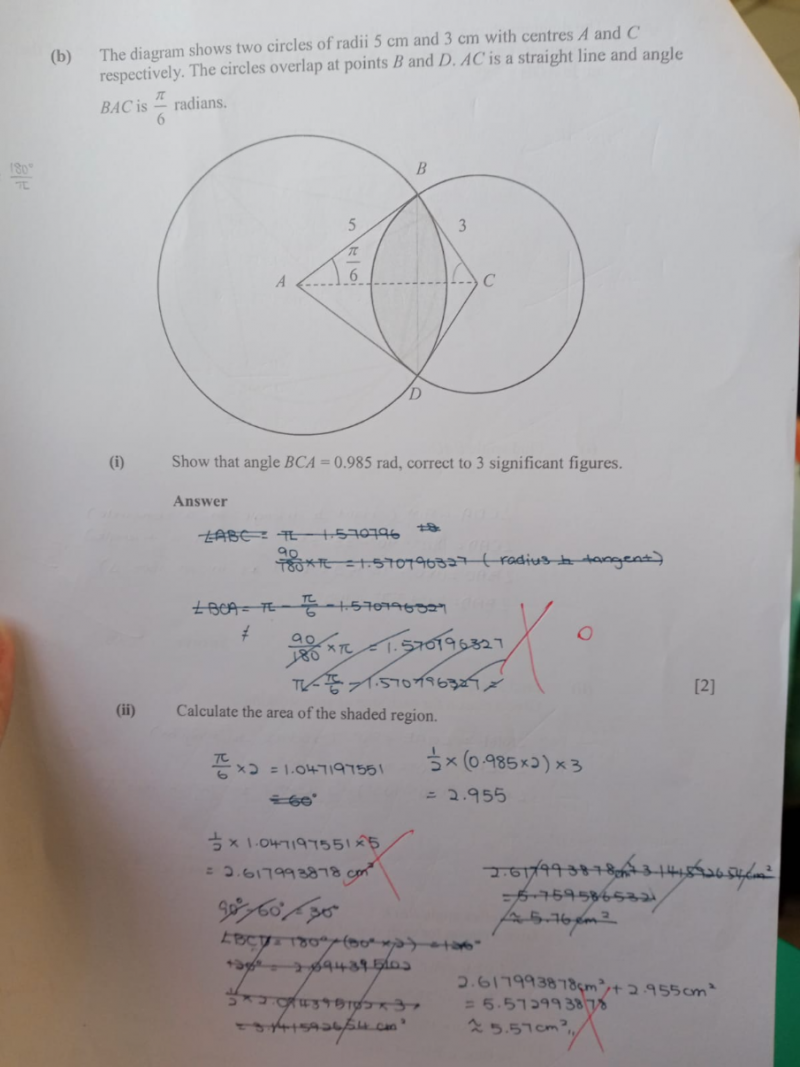# QuestionThank you

(a)  Let the midpoint of BD be O.

BO/5 = sin (π/6

BO = 5 x (1/2) = (5/2)

sin (BCA) =  (5/2)  / 3 = (5/6)

BCA = sin -1 (5/6) = 0.985 rad   (3 s.f.)

(b)   Area of shaded region =

(Area of Sector ABD – Area of Triangle ABD) + (Area of Sector BCD – Area of Triangle BCD)

Area of Triangle ABD + Area of Triangle BCD =  Area of Triangle ABC x 2

Let’s work on half the area of the shaded region and then x 2 later.

Half area of sector ABD Area =  (5)(5)(π)  / 360 x  (30)         [π/6 radians = 300 ]

= 6.545

Half area of sector BCD = (3)(3)(π)  / 6.28 x (0.985)    [360 = 6.28 radians ]

= 4.435

Total of half areas of sector ABD and BCD = 6.545 + 4.435 = 10.98

AO/5 = cos (π/6 ) = 0.866  => AO = 5 x 0.866 = 4.33

OC/3 = cos (0.985 rad) = 0.553 => OC = 3 x 0.553 = 1.659

AC = 4.33+1.659 =  5.989

Area of Triangle ABC  = (1/2) (AC) (BO)   = (1/2) (5.989)(5/2) = 7.486

Area of half shaded region = 10.98 – 7.486 = 3.494

Total area of shaded region = 3.494 x 2 = 6.99 cm ( to 3 s.f)

0 Replies 1 Like ✔Accepted Answer